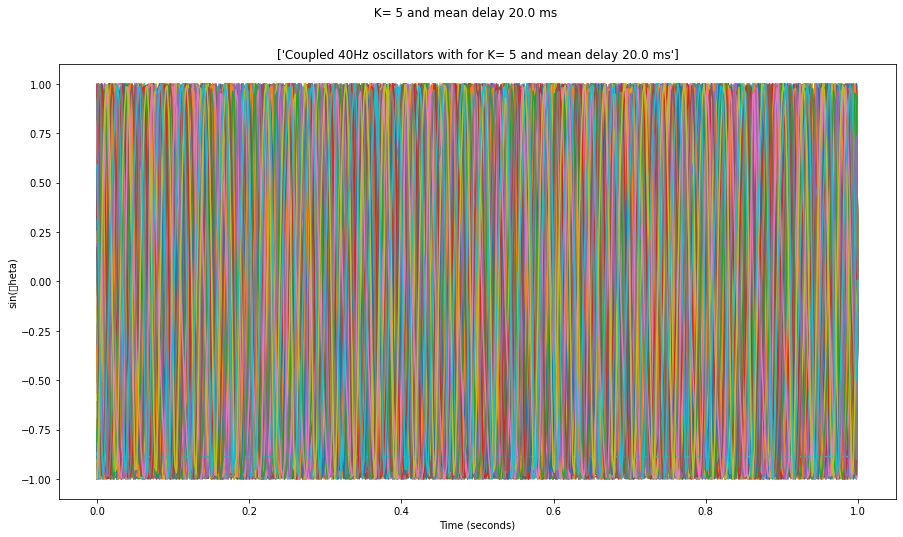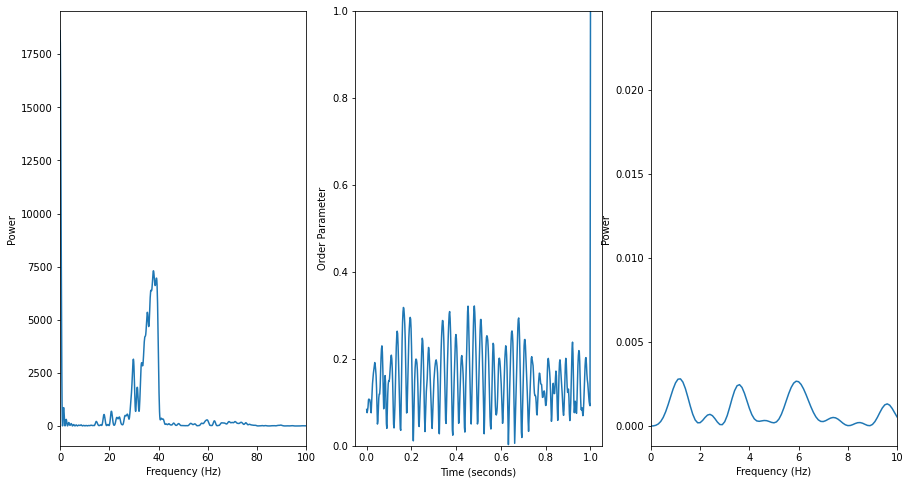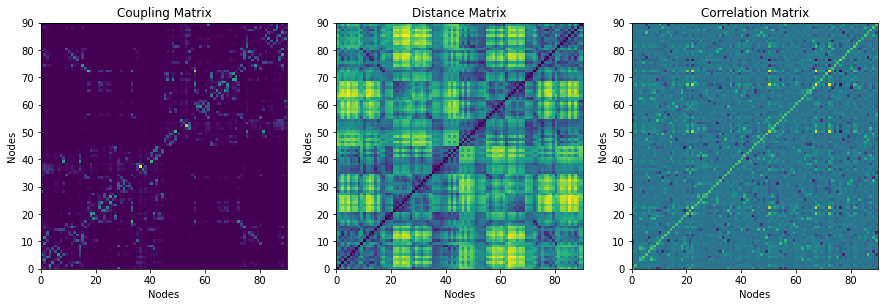# Kuramoto model

The goal here is to re-implement the Kuramoto model, following a lecture from Joana Cabral and the code that is provided, but using python instead of matlab.

Let's first initialize the notebook:

In :
from __future__ import division, print_function
import numpy as np
np.set_printoptions(precision=6, suppress=True)
%matplotlib inline
import matplotlib.pyplot as plt
fig_width = 10
figsize = (fig_width, fig_width)


### initialize simulations¶

In :
#####################%%%%%%
#
# Code to call simulations of a network model
# and visualize the results.
#
# Generates figure with:
# - timeseries, power spectra and phase order over time
# - matrices of coupling wights, distances and 'Functional Connectivity'
#
# Joana Cabral January 2019 joanacabral@med.uminho.pt
#
#####################%%%%%%

import numpy as np
# Define here the Network Spatial and Temporal structure

# Define the Structural Network as a NxN matrix

fname = '/tmp/AAL_matrices.mat'

# Number of coupled Units
N = C.shape

# note: using the line below Normalizes the whole matrix by the mean
# of all non-diagonal elements is 1.
# C=C/mean(C(ones(N)-eye(N)>0));
# here I do what you describe in the following line:
# Normalize such that the mean of all non-diagonal elements is 1.
C[np.diag(np.ones(N))==0] /= C[np.diag(np.ones(N))==0].mean()

# Distance between areas
D /= 1000 # Distance matrix in meters

# Define here the parameters of the network model to be manipulated

# Node natural frequency in Hz
f = 40    # (i.e. f=40)):

# Mean Delay in seconds
MD = 0.02 # (i.e. MD = 0.01)

# Global Coupling strength
K = 5 #


### Kuramoto model: Process the simulated data and generate figures¶

In :
# Call here the function of the Network Model
import numpy as np
def Kuramoto_Delays_Run_AAL(C, D, f, K, MD):
############################%%%#
#
# Function to run simulations of coupled systems using the
# KURAMOTO MODEL OF COUPLED OSCILLATORS WITH TIME DELAYS
#
# - Each node is represented by a Phase Oscillator
# - with Natural Frequency f
# - coupled according to a  connectivity matrix C
# - with a distance matrix D (scaled to delays by the mean delay MD)
#
# All units in Seconds, Meters, Hz
#
# Simulation code written by Joana Cabral,
# January 2019, joana.cabral@psych.ox.ac.uk
#
##############%%%%%#

# MODEL PARAMETERS

# # Define simulation Parameters
# f=40 # Node natural frequency in Hz (i.e. f=40))
# MD=0.02 # Mean Delay in seconds (i.e. MD=0.01)
# K=5;
dt = 1.e-4 # Resolution of model integration in seconds (i.e. dt=1e-4)
tmax = 1. # Total simulation time in seconds
t_prev = 0. # Total preview time in seconds
dt_save = 2.e-3 # Resolution of saved simulations in seconds
noise = 0

# Normalize parameters
N = C.shape # Number of units
Omegas = 2*np.pi*f*np.ones(N)*dt # Frequency of all units in radians/second
kC = K*C*dt # Scale matrix C with 'K' and 'dt' to avoid doing it at each step
dsig = np.sqrt(dt)*noise # normalize std of noise with dt

# Set a matrix of Delays containing the number of time-steps between nodes
# Delays are integer numbers, so make sure the dt is much smaller than the
# smallest delay.
if MD==0:
Delays = np.zeros_like(C) # Set all delays to 0.
else:
Delays = D / D[C>0].mean() * MD / dt
Delays = Delays.astype(np.int)

Delays[C==0] = 0.

Max_History = np.max(Delays.ravel()) + 1

# Revert the Delays matrix such that it contains the index of the History
# that we need to retrieve at each dt
Delays = Max_History - Delays

# Initialization

# History of Phases is needed at dt resolution for as long as the longest
# delay. The system is initialized all desinchronized and uncoupled
# oscillating at their natural frequencies

Phases_History = 2*np.pi*np.random.rand(N, Max_History)
Phases_History += Omegas[:, None] #* np.ones(N, Max_History)#*np.arange(Max_History)[:, None]
Phases_History = np.mod(Phases_History, 2*np.pi) # all values between 0 and 2pi

# This History matrix will be continuously updated (sliding history).
# figure plot(sin(Phases_History'))   # To see the History

# Simulated activity will be saved only at dt_save resolution
Phases_Save = np.zeros((N, int(tmax/dt_save)))
sumz = np.zeros(N)

# Run simulations
print('Now running for K=',  K,  ', mean Delay = ', MD*1e3, 'ms')
print('Max_History=',  Max_History, 'steps')

import time
tic = time.time() # to count the time of each run
nt = 0
import tqdm
for i_t in tqdm.tqdm(range(int((t_prev+tmax)/dt))):
# We only start saving after t_prev
Phase_Now = Phases_History[:, -1] # The last collumn of Z is 'now'
# Input from coupled units
for n in range(N):
sumzn = 0 # Intitalize total coupling received into node n
for p in range(N):
if kC[n, p]>0: # Calculate only input from connected nodes (faster)
phase = Phases_History[p, Delays[n, p]]-Phase_Now[n]
sumzn += kC[n,p] * np.sin(phase)
sumz[n] = sumzn

if MD>0: # Slide History only if the delays are >0
Phases_History[:, :-2] = Phases_History[:, 1:-1]

# Update the end of History with evolution in the last time step
Phases_History[:, -1] = Phase_Now
Phases_History[:, -1] += Omegas + sumz + dsig*np.random.randn(N)

# Save dt_save resolution after t_prev
if np.mod(i_t, int(dt_save/dt)) == 0 and i_t*dt>t_prev:
Phases_Save[:, nt] = Phases_History[:, -1]
nt += 1

toc = time.time() - tic
print('Finished, lasted %.3f ' % toc , ' secs for real %.3f ', t_prev+tmax , ' seconds')

print('Simu speed ratio %.3f =' % (toc/(t_prev+tmax)), ' Realseconds/SimuSecond')

return Phases_Save, dt_save

In :
Phases_Save, dt_save = Kuramoto_Delays_Run_AAL(C, D, f, K, MD)

  0%|          | 11/10000 [00:00<01:37, 102.94it/s]
Now running for K= 5 , mean Delay =  20.0 ms
Max_History= 498 steps

100%|██████████| 10000/10000 [01:32<00:00, 107.64it/s]
Finished, lasted 92.920   secs for real %.3f  1.0  seconds
Simu speed ratio 92.920 =  Realseconds/SimuSecond


In :
%whos

Variable                  Type        Data/Info
-----------------------------------------------
C                         ndarray     90x90: 8100 elems, type float64, 64800 bytes
D                         ndarray     90x90: 8100 elems, type float64, 64800 bytes
K                         int         5
Kuramoto_Delays_Run_AAL   function    <function Kuramoto_Delays<...>un_AAL at 0x7f8db86901f0>
MD                        float       0.02
N                         int         90
Phases_Save               ndarray     90x500: 45000 elems, type float64, 360000 bytes (351.5625 kb)
division                  _Feature    _Feature((2, 2, 0, 'alpha<...>, 0, 'alpha', 0), 131072)
dt_save                   float       0.002
f                         int         40
fig_width                 int         10
figsize                   tuple       n=2
fname                     str         /tmp/AAL_matrices.mat
np                        module      <module 'numpy' from '/us<...>kages/numpy/__init__.py'>
plt                       module      <module 'matplotlib.pyplo<...>es/matplotlib/pyplot.py'>
print_function            _Feature    _Feature((2, 6, 0, 'alpha<...> 0, 'alpha', 0), 1048576)

In :
%matplotlib inline

In :
# Process the simulated data and generate figures
import matplotlib.pyplot as plt

N_time = Phases_Save.shape
tmax = N_time * dt_save
time = np.linspace(0, tmax, N_time)

# Plot simulated time series
fig, ax = plt.subplots(1, 1, figsize=(15, 8))
ax.plot(time, np.sin(Phases_Save.T))
ax.set_xlabel('Time (seconds)')
ax.set_ylabel('sin(\theta)')
ax.set_title(['Coupled ' + str(f) + 'Hz oscillators with for K= ' + str(K) + ' and mean delay ' + str(MD*1e3) + ' ms'])
fig.suptitle(' K= ' + str(K) + ' and mean delay ' + str(MD*1e3) + ' ms');

/usr/local/anaconda3/envs/sim/lib/python3.9/site-packages/matplotlib/backends/backend_agg.py:238: RuntimeWarning: Glyph 9 missing from current font.
font.set_text(s, 0.0, flags=flags)
/usr/local/anaconda3/envs/sim/lib/python3.9/site-packages/matplotlib/backends/backend_agg.py:201: RuntimeWarning: Glyph 9 missing from current font.
font.set_text(s, 0, flags=flags)In :
# Power Spectrum
fig, axs = plt.subplots(1, 3, figsize=(15, 8))
fbins = 5000
freqZ = np.arange(fbins)/(dt_save*fbins)
ind100Hz = np.argmin(freqZ==100)
Fourier = np.fft.fft(np.sin(Phases_Save), n=fbins, axis=-1)
PSD = np.abs(Fourier)**2
ax = axs
ax.plot(freqZ[:fbins//2], PSD[:, :fbins//2].mean(axis=0))
ax.set_xlim([0, 100])
ax.set_xlabel('Frequency (Hz)')
ax.set_ylabel('Power')

# Plot the Order Parameter over time
ax = axs
OP = np.abs(np.mean(np.exp(1j*Phases_Save), axis=0))
ax.plot(time, OP)
ax.set_ylim([0, 1])
ax.set_xlabel('Time (seconds)')
ax.set_ylabel('Order Parameter')

# Power Spectrum of the Order Parameter
ax = axs
Fourier = np.fft.fft(OP-OP.mean(), n=fbins, axis=0)
PSD = np.abs(Fourier)**2
PSD = PSD[:fbins//2]
PSD /= PSD.sum()
ax.plot(freqZ[:fbins//2], PSD)
ax.set_xlim([0, 10])
ax.set_xlabel('Frequency (Hz)')
ax.set_ylabel('Power');In :
# Plot Connectivity and Distance Matrices and mean Phase Coherence

# Change the order of units for visualization (optional)
Order = np.arange(0, N, 2) # This sorts the AAL areas into left and right hemispheres
Order = np.hstack((Order, np.arange(N-1, 0, -2)))

fig, axs = plt.subplots(1, 3, figsize=(15, 8))
ax = axs
# colormap(jet)
ax.pcolormesh(C[Order, :][:, Order])

ax.set_xlabel('Nodes')
ax.set_ylabel('Nodes')
ax.set_title('Coupling Matrix')
ax.axis('square')
#plt.colorbar()

ax = axs
ax.pcolormesh(D[Order, :][:, Order])
ax.set_xlabel('Nodes')
ax.set_ylabel('Nodes')
ax.set_title('Distance Matrix')
ax.axis('square')
#plt.colorbar()

ax = axs
X = np.sin(Phases_Save)
FC = (X[:, None, :] * X[None, :, :]).mean(axis=-1)
ax.pcolormesh(FC[Order, :][:, Order])
ax.set_xlabel('Nodes')
ax.set_ylabel('Nodes')
ax.set_title('Correlation Matrix')
ax.axis('square');
#plt.colorbar()